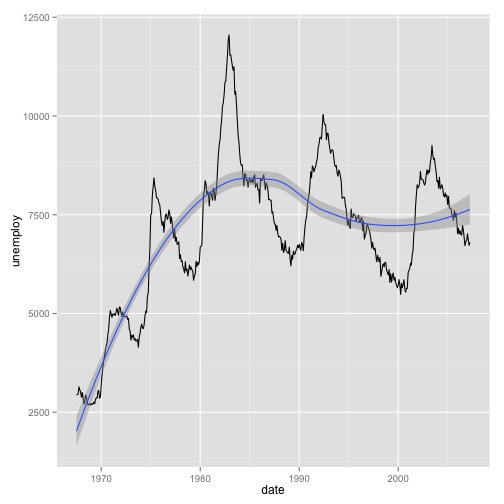I gave a short talk today to the [Davis R Users’ Group] about ggplot. This what I presented. Additional resources at the bottom of this post

`ggplot` is an R package for data exploration and producing plots. It produces fantastic-looking graphics and allows one to slice and dice one’s data in many different ways.

### Comparing with base graphics

(This example from Stack Overflow)

First, get the package:

``````install.packages("ggplot2")
``````
``````library(ggplot2)
``````

Let’s say we wanted to plot some two-variable data, changing color and shape by the sub-category of data. Here’s a data set:

``````data.df <- data.frame(Plant = c("Plant1", "Plant1", "Plant1", "Plant2", "Plant2",
"Plant2"), Type = c(1, 2, 3, 1, 2, 3), Axis1 = c(0.2, -0.4, 0.8, -0.2, -0.7,
0.1), Axis2 = c(0.5, 0.3, -0.1, -0.3, -0.1, -0.8))
``````

Using normal R graphics, we might do this:

``````color_foo <- colorRampPalette(c("lightblue", "darkblue"))
colors <- color_foo(3)

plot(range(data.df[, 3]), range(data.df[, 4]), xlab = "Axis 1", ylab = "Axis 2",
type = "n")
points(data.df\$Axis1, data.df\$Axis2, pch = c(1, 2)[data.df\$Plant], col = colors[data.df\$Type])
legend("topright", legend = c("Plant1", "Plant2"), pch = 1:2)
legend("bottomright", legend = c("Type1", "Type2", "Type3"), pch = 20, col = colors)
``````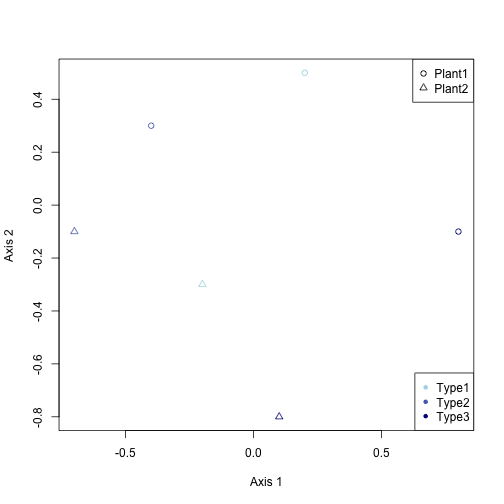With `ggplot`, you just do this:

``````ggplot(data.df, aes(x = Axis1, y = Axis2, shape = Plant, color = Type)) + geom_point(size = 5)
``````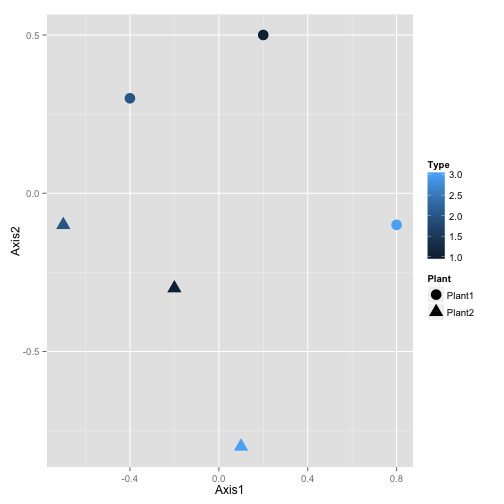And it looks much better!

But `ggplot()` really shines when you have a lot of data. Here’s an example of some fish survey data that I produced with it: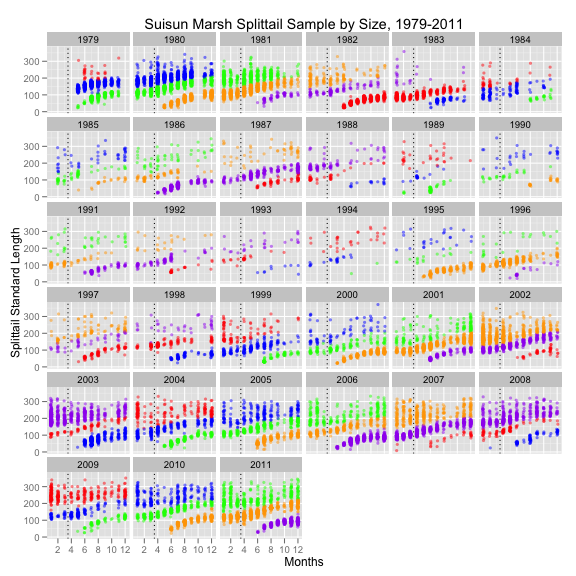### Tutorial

`ggplot` is best used on data in data frame form. Let’s look at a data set already in R, this about the sleep habits of different animal species

``````head(msleep)
``````
``````##                         name      genus  vore        order conservation
## 1                    Cheetah   Acinonyx carni    Carnivora           lc
## 2                 Owl monkey      Aotus  omni     Primates         <NA>
## 3            Mountain beaver Aplodontia herbi     Rodentia           nt
## 4 Greater short-tailed shrew    Blarina  omni Soricomorpha           lc
## 5                        Cow        Bos herbi Artiodactyla domesticated
## 6           Three-toed sloth   Bradypus herbi       Pilosa         <NA>
##   sleep_total sleep_rem sleep_cycle awake brainwt  bodywt
## 1        12.1        NA          NA  11.9      NA  50.000
## 2        17.0       1.8          NA   7.0 0.01550   0.480
## 3        14.4       2.4          NA   9.6      NA   1.350
## 4        14.9       2.3      0.1333   9.1 0.00029   0.019
## 5         4.0       0.7      0.6667  20.0 0.42300 600.000
## 6        14.4       2.2      0.7667   9.6      NA   3.850
``````

Now, lets start with a basic plot. Let’s create a scatterplot of body weight against total hours sleep:

``````a <- ggplot(data = msleep, aes(x = bodywt, y = sleep_total))
a <- a + geom_point()
a <- a + xlab("Body Weight") + ylab("Total Hours Sleep") + ggtitle("Some Sleep Data")
a
``````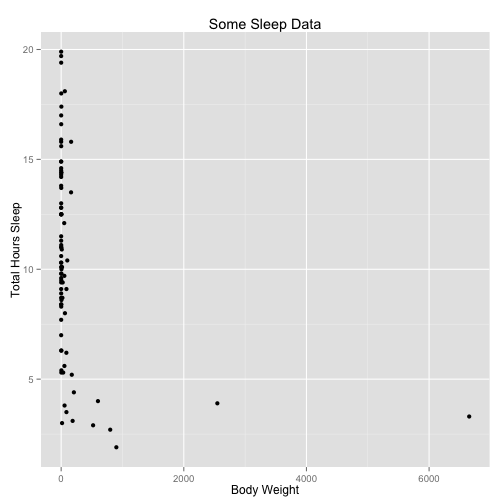Let’s parse what we just did. The `ggplot()` command creates a plot object. In it we assigned a data set. `aes()` creates what Hadley Wickham calls an aesthetic: a mapping of variables to various parts of the plot.

We then add components to the plot. `geom_point()` adds a layer of points, using the base aesthetic mapping. The third line adds labels. Typing the variable name `a` displays the plot. Alternately, one can use the command `ggsave()` to save the plot as a file, as in

``````ggsave("sleepplot.png", plot = a, width = 4, height = 4)
``````

Now, one of the great things we can do with `ggplot` is slice the data different ways. For instance, we can plot another variable against color:

``````a <- ggplot(data = msleep, aes(x = bodywt, y = sleep_total, col = vore))
a <- a + geom_point()
a <- a + xlab("Body Weight") + ylab("Total Hours Sleep") + ggtitle("Some Sleep Data")
a
``````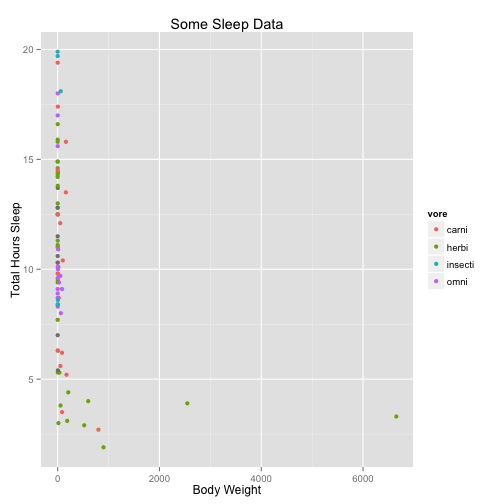You can also use map size and alpha (transparency) to variables.

We can transform variables directly in the `ggplot` call, as well:

``````a <- ggplot(data = msleep, aes(x = log(bodywt), y = sleep_rem/sleep_total, col = vore))
a <- a + geom_point()
a <- a + xlab("Log Body Weight") + ylab("Fraction of Sleep that is REM") + ggtitle("Some Sleep Data") +
scale_color_discrete(name = "Trophic Level")
a
``````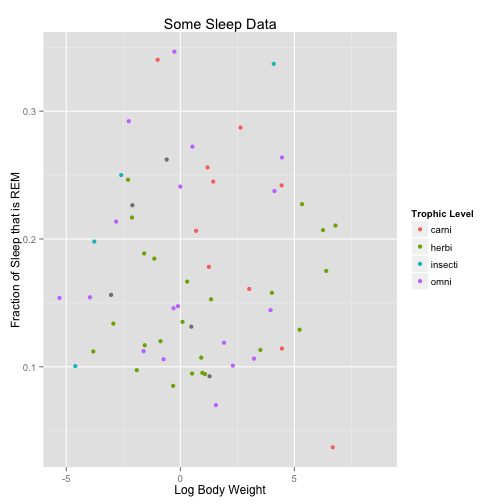Within the `geom` calls, we can change plotting options

``````a <- ggplot(data = msleep, aes(x = log(bodywt), y = sleep_rem/sleep_total, col = vore))
a <- a + geom_point(size = 5)
a <- a + xlab("Log Body Weight") + ylab("Fraction of Sleep that is REM") + ggtitle("Some Sleep Data") +
scale_color_discrete(name = "Trophic Level")
a
``````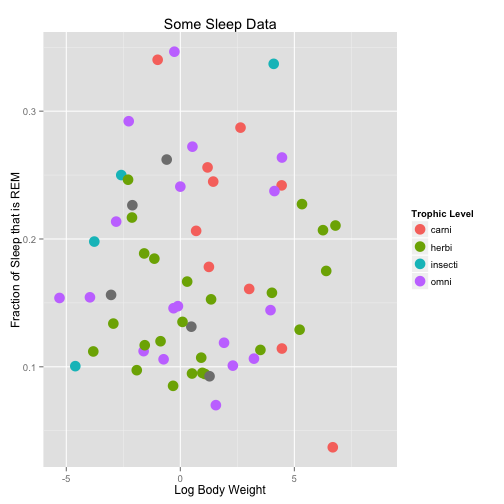Another way to split up the way we look at data is with facets. These break up the plot into multiple plots. If you are splitting the plot up by one variable, use `facet_wrap`. If you are using two variables, use `facet_grid`.

``````a <- ggplot(data = msleep, aes(x = log(bodywt), y = sleep_rem/sleep_total))
a <- a + geom_point(size = 5)
a <- a + facet_wrap(~vore)
a <- a + xlab("Log Body Weight") + ylab("Fraction of Sleep that is REM") + ggtitle("Some Sleep Data")
a
``````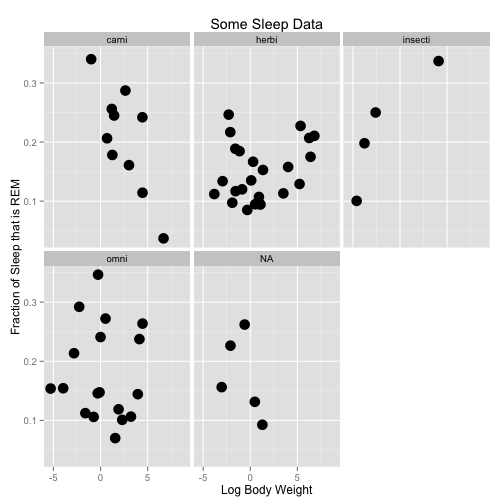``````a <- ggplot(data = msleep, aes(x = log(bodywt), y = sleep_rem/sleep_total))
a <- a + geom_point(size = 2)
a <- a + facet_grid(conservation ~ vore)
a <- a + xlab("Log Body Weight") + ylab("Fraction of Sleep that is REM") + ggtitle("Some Sleep Data")
a
``````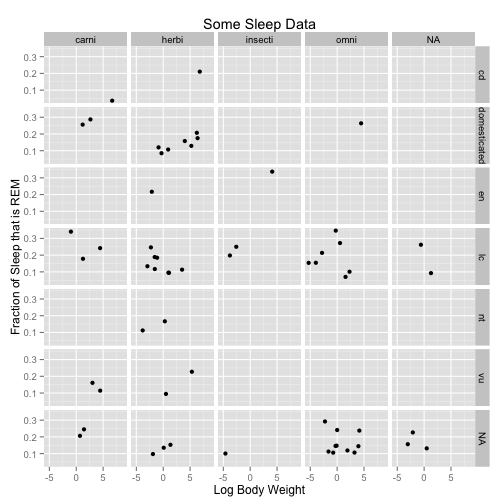Let’s use a different data set to so line plots:

``````head(economics)
``````
``````##         date   pce    pop psavert uempmed unemploy
## 1 1967-06-30 507.8 198712     9.8     4.5     2944
## 2 1967-07-31 510.9 198911     9.8     4.7     2945
## 3 1967-08-31 516.7 199113     9.0     4.6     2958
## 4 1967-09-30 513.3 199311     9.8     4.9     3143
## 5 1967-10-31 518.5 199498     9.7     4.7     3066
## 6 1967-11-30 526.2 199657     9.4     4.8     3018
``````
``````a <- ggplot(data = economics, aes(x = date, y = unemploy))
a <- a + geom_line()
a
``````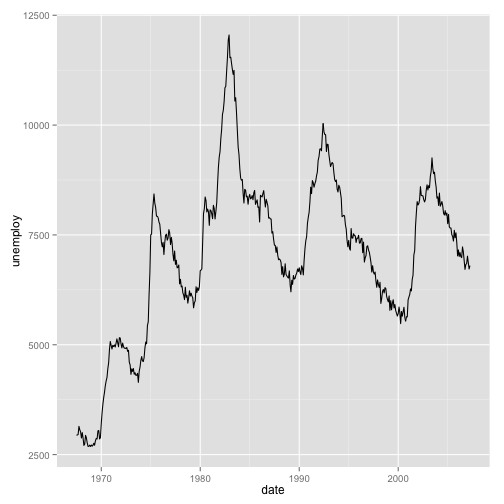We can add statistical transformations to this series, for instance:

``````a <- ggplot(data = economics, aes(x = date, y = unemploy))
a <- a + geom_line()
a <- a + geom_smooth()
a
``````
``````## geom_smooth: method="auto" and size of largest group is <1000, so using
## loess. Use 'method = x' to change the smoothing method.
``````